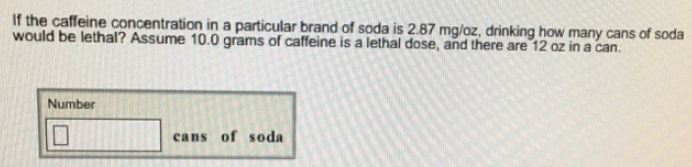# Problem: If the caffeine concentration in a particular brand of soda is 2.87 mg/oz, drinking how many cans of soda would be lethal? Assume 10.0 grams of caffeine is a lethal dose, and there are 12 oz in a can.

###### FREE Expert Solution

BY converting grams of lethal dose to milligrams, we can find the number of cans needed to drink to be lethal

86% (366 ratings)###### Problem Details

If the caffeine concentration in a particular brand of soda is 2.87 mg/oz, drinking how many cans of soda would be lethal? Assume 10.0 grams of caffeine is a lethal dose, and there are 12 oz in a can.